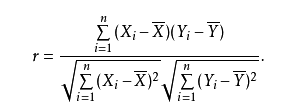• from scipy.stats import pearsonr x = [0.5, 0.4, 0.6, 0.3, 0.6, 0.2, 0.7, 0.5] y = [0.6, 0.4, 0.4, 0.3, 0.7, 0.2, 0.5, 0.6] print(pearsonr(x, y)) ...# r:相关系数[-1，1]之间 # p:p值越小  ...
from scipy.stats import pearsonr

x = [0.5, 0.4, 0.6, 0.3, 0.6, 0.2, 0.7, 0.5]
y = [0.6, 0.4, 0.4, 0.3, 0.7, 0.2, 0.5, 0.6]
print(pearsonr(x, y))

# 输出:(r, p)
# r:相关系数[-1，1]之间
# p:p值越小


展开全文• Python三种方法计算皮尔逊相关系数（Pearson correlation coefficient） 0 皮尔逊系数 1 python计算方法 1.1 根据公式手写 1.2 numpy的函数 1.3 scipy.stats中的函数 0 皮尔逊系数  在统计学中，皮尔逊相关系数( ...
Python三种方法计算皮尔逊相关系数（Pearson correlation coefficient）
0 皮尔逊系数
1 python计算方法
1.1 根据公式手写
1.2 numpy的函数
1.3 scipy.stats中的函数
0 皮尔逊系数
在统计学中，皮尔逊相关系数( Pearson correlation coefficient），又称皮尔逊积矩相关系数（Pearson product-moment correlation coefficient，简称 PPMCC或PCCs）。用于衡量两个变量X和Y之间的线性相关相关关系，值域在-1与1之间。1 python计算方法
笔者发现了三种方式，用户可根据自身需求进行使用或者比对：
1.1 根据公式手写
def cal_pccs(x, y, n):
“”"
warning: data format must be narray
:param x: Variable 1
:param y: The variable 2
:param n: The number of elements in x
:return: pccs
“”"
sum_xy = np.sum(np.sum(xy))
sum_x = np.sum(np.sum(x))
sum_y = np.sum(np.sum(y))
sum_x2 = np.sum(np.sum(xx))
sum_y2 = np.sum(np.sum(yy))
pcc = (nsum_xy-sum_xsum_y)/np.sqrt((nsum_x2-sum_xsum_x)(nsum_y2-sum_ysum_y))
return pcc
1.2 numpy的函数
pccs = np.corrcoef(x, y)
1.3 scipy.stats中的函数
from scipy.stats import pearsonr
pccs = pearsonr(x, y)


展开全文• pearson相关系数：用于判断...Python计算pearson相关系数：1. 使用numpy计算(corrcoef)，以下是先标准化再求相关系数importnumpy as npimportpandas as pdaa= np.array([2,3,9,6,8])bb= np.array([5,6,3,7,9])cc=n...
pearson相关系数：用于判断数据是否线性相关的方法。注意：不线性相关并不代表不相关，因为可能是非线性相关。Python计算pearson相关系数：1. 使用numpy计算(corrcoef)，以下是先标准化再求相关系数importnumpy as npimportpandas as pdaa= np.array([2,3,9,6,8])bb= np.array([5,6,3,7,9])cc=np.array([aa, bb])print(cc)cc_mean= np.mean(cc, axis=0)  #axis=0,表示按列求均值 ——— 即第一维cc_std = np.std(cc, axis=0)cc_zscore= (cc-cc_mean)/cc_std   #标准化cc_zscore_corr= np.corrcoef(cc_zscore)  #相关系数矩阵print(cc_zscore_corr)其中：def corrcoef(x, y=None, rowvar=True, bias=np._NoValue, ddof=np._NoValue):"""Return Pearson product-moment correlation coefficients.Please refer to the documentation for cov for more detail.  Therelationship between the correlation coefficient matrix, R, and thecovariance matrix, C, is.. math::R_{ij} = \\frac{ C_{ij} } { \\sqrt{ C_{ii} * C_{jj} } }The values of R are between -1 and 1, inclusive.Parameters----------x : array_likeA 1-D or 2-D array containing multiple variables and observations.Each row of x represents a variable, and each column a singleobservation of all those variables. Also see rowvar below.2. 使用pandas计算相关系数cc_pd = pd.DataFrame(cc_zscore.T, columns=['c1', 'c2'])cc_corr= cc_pd.corr(method='spearman')   #相关系数矩阵其中，method中，有三种相关系数的计算方式，包括 —— 'pearson', 'kendall', 'spearman'，常用的是线性相关pearson。Parameters----------method : {'pearson', 'kendall', 'spearman'}*pearson : standard correlation coefficient*kendall : Kendall Tau correlation coefficient* spearman : Spearman rank correlationcc_corr 值可用于获取：某个因子与其他因子的相关系数：print(cc_corr['c1'])  #某个因子与其他因子的相关系数附：pandas计算协方差print(cc_pd.c1.cov(cc_pd.c2))   #协方差print(cc_pd.c1.corr(cc_pd.c2))  #两个因子的相关系数y_cov = cc_pd.cov()     #协方差矩阵3. 可直接计算，因为pearson相关系数的计算公式为：cov(X,Y)表示的是协方差var(x)和var(y)表示的是方差##参考：
展开全文• 1、皮尔逊相关系数在统计学中，皮尔逊相关系数( Pearson correlation coefficient)，又称皮尔逊积矩相关系数(Pearson product-moment correlation coefficient，简称 PPMCC或PCCs)。用于衡量两个变量X和Y之间的线性...
1、皮尔逊相关系数在统计学中，皮尔逊相关系数( Pearson correlation coefficient)，又称皮尔逊积矩相关系数(Pearson product-moment correlation coefficient，简称 PPMCC或PCCs)。用于衡量两个变量X和Y之间的线性相关相关关系，值域在-1与1之间。2、根据公式手写import numpy as npimport pandas as pdfrom math import *def multipl(a,b):sumofab=0.0for i in range(len(a)):temp=a[i]*b[i]sumofab+=tempreturn sumofabdef cal_pccs(x,y):n=len(x)#求和sum1=sum(x)sum2=sum(y)#求乘积之和sumofxy=multipl(x,y)#求平方和sumofx2 = sum([pow(i,2) for i in x])sumofy2 = sum([pow(j,2) for j in y])num=sumofxy-(float(sum1)*float(sum2)/n)#计算皮尔逊相关系数den=sqrt((sumofx2-float(sum1**2)/n)*(sumofy2-float(sum2**2)/n))return num/denpct_chg = pd.Series({'000001.SZ':-1.7391, '000002.SZ':0.6250,'000004.SZ':1.1378,'002600.SZ':0.0000,'000010.SZ':-1.0013})vol = pd.Series({'000001.SZ':249326.31,'000002.SZ':338224.97,'000004.SZ':211876.00,'000010.SZ':222782.00,'002600.SZ':342096.76})print(cal_pccs(pct_chg, vol))3、talib的函数import talib as taimport pandas as pdpct_chg = pd.Series({'000001.SZ':-1.7391, '000002.SZ':0.6250,'000004.SZ':1.1378,'002600.SZ':0.0000,'000010.SZ':-1.0013})vol = pd.Series({'000001.SZ':249326.31,'000002.SZ':338224.97,'000004.SZ':211876.00,'000010.SZ':222782.00,'002600.SZ':342096.76})print(ta.CORREL(pct_chg,vol,5)[-1])4、numpy的函数import numpy as npimport pandas as pdpct_chg = pd.Series({'000001.SZ':-1.7391, '000002.SZ':0.6250,'000004.SZ':1.1378,'002600.SZ':0.0000,'000010.SZ':-1.0013})vol = pd.Series({'000001.SZ':249326.31,'000002.SZ':338224.97,'000004.SZ':211876.00,'000010.SZ':222782.00,'002600.SZ':342096.76})pccs = np.corrcoef(pct_chg, vol)print(pccs)5、scipy的函数import numpy as npimport pandas as pdfrom scipy.stats import pearsonrpct_chg = pd.Series({'000001.SZ':-1.7391, '000002.SZ':0.6250,'000004.SZ':1.1378,'002600.SZ':0.0000,'000010.SZ':-1.0013})vol = pd.Series({'000001.SZ':249326.31,'000002.SZ':338224.97,'000004.SZ':211876.00,'000010.SZ':222782.00,'002600.SZ':342096.76})pccs = pearsonr(pct_chg, vol)print(pccs)- END -转载：感谢您对张伟江个人博客网站平台的认可，以及对我们原创作品以及文章的青睐，非常欢迎各位朋友分享到个人站长或者朋友圈，但转载请说明文章出处“来源张伟江个人博客”。
展开全文• 数据探索 计算相关系数 为了更加准确地描述变量之间的线性相关...皮尔逊相关系数（Pearson Correlation Coefficient） 一般用于分析两个连续性变量之间的关系，其计算公式如下。 r=∑i=1n(xi−x‾)(yi−y‾)∑i=1n(x...
• 今天小编就为大家分享一篇Python 余弦相似度与皮尔逊相关系数 计算实例，具有很好的参考价值，希望对大家有所帮助。一起跟随小编过来看看吧
• pearson相关系数：用于判断...Python计算pearson相关系数：1. 使用numpy计算(corrcoef)，以下是先标准化再求相关系数importnumpy as npimportpandas as pdaa= np.array([2,3,9,6,8])bb= np.array([5,6,3,7,9])cc=n...
• 在研究脑网络时，看到了用皮尔逊相关系数计算脑功能网络的，就简单的学了一下它的计算 这里用到的是Python的numpy库 首先，最前面要加import，先导入这个模块 import numpy as np 然后，调用corrcoef() 其中data...numpy
• 1.常见理解误区(1)计算出变量A和变量B的皮尔逊相关系数为0，不代表A和B之间没有相关性，只能说明A和B之间不存在线性相关关系。例：温度和冰淇淋销量之间的散点图像如下，可以发现大致成二次函数图像，随着温度升高，...
• 文章目录0 皮尔逊系数1 python计算... 在统计学中，皮尔逊相关系数( Pearson correlation coefficient），又称皮尔逊积矩相关系数（Pearson product-moment correlation coefficient，简称 PPMCC或PCCs）。用于衡量...
• 源代码不记得是哪里获取的了，侵删。... #计算皮尔逊相关系数 den=sqrt((sumofx2-float(sum1**2)/n)*(sumofy2-float(sum2**2)/n)) return num/den 转载于:https://www.cnblogs.com/IvyWong/p/9482687.html
• d=1-pdist([x,x],'cosine') 皮尔逊相关系数(Pearson correlation) (1) 皮尔逊相关系数的定义 前面提到的余弦相似度只与向量方向有关，但它会受到向量的平移影响，在夹角余弦公式中如果将 x 平移到 x+1, 余弦值就会...
• 查了一下，发现可以通过皮尔逊相关系数和spearman相关系数来计算特征之间的相似度，下面将介绍这两个系数和区别。 皮尔逊相关系数 下面是皮尔逊相关系数的计算公式，只需要将（X和Y的协方差）/（X的标准差*Y的标准...特征工程 机器学习
• 1.常见理解误区（1）计算出变量A和变量B的皮尔逊相关系数为0，不代表A和B之间没有相关性，只能说明A和B之间不存在线性相关关系。例：温度和冰淇淋销量之间的散点图像如下，可以发现大致成二次函数图像，随着温度升高...
• 皮尔逊相关系数具有平移不变性和尺度不变性，计算出了两个向量（维度）的相关性。  在python中的实现：' import numpy as np x=np.random.random(10) y=np.random.random(10) #方法一：根据公式求解 x_=x-...
• 在统计学中，皮尔逊相关系数( Pearson correlation coefficient），又称皮尔逊积矩相关系数（Pearson product-moment correlation coefficient，简称 PPMCC或PCCs）。用于衡量两个变量X和Y之间的线性相关相关关系，...1024程序员节
• 皮尔逊相关系数 定义+python代码实现 （与王印讨论公式） 标签： python相关性系数皮尔逊系数 2016-10-25 20:59 153人阅读 评论(0) 收藏 举报  分类： 集体智慧编程 版权声明：本文为...算法 数据结构
• 皮尔逊相关系数的计算(python代码版) 1 2 3 4 5 6 7 8 9 10 11 12 13 14 15 16 17 18 19 20 21 22 23 24 25 ...算法 C 数据结构 matlab
• 1.常见理解误区（1）计算出变量A和变量B的皮尔逊相关系数为0，不代表A和B之间没有相关性，只能说明A和B之间不存在线性相关关系。例：温度和冰淇淋销量之间的散点图像如下，可以发现大致成二次函数图像，随着温度升高...
• 概念介绍寻找相似K线是投资者用来研究“历史是否总会重演”的常用方法，目前许多交易工具都已经提供搜索相似K线功能。...相似度匹配进行相似度匹配时我们使用“皮尔逊相关系数”（Pearson product-mome...
• 基于皮尔逊相关系数的用户相似推荐算法python实现 随着电子商务规模的不断扩大，商品个数和种类快速增长，顾客需要花费大量的时间才能找到自己想买的商品。这种浏览大量无关的信息和产品过程无疑会使淹没在信息过载...推荐系统
• #皮尔逊相关系数： 用于度量两个变量X和Y之间的相关（线性相关），其值介于-1与1之间 from scipy.stats import pearsonr x = [0.5, 0.4, 0.6, 0.3, 0.6, 0.2, 0.7, 0.5] y = [0.6, 0.4, 0.4, 0.3, 0.7, 0.2...
• d=1-pdist([x,x],'cosine') 皮尔逊相关系数(Pearson correlation) (1) 皮尔逊相关系数的定义 前面提到的余弦相似度只与向量方向有关，但它会受到向量的平移影响，在夹角余弦公式中如果将 x 平移到 x+1, 余弦值就会......

# python皮尔逊相关系数python 订阅